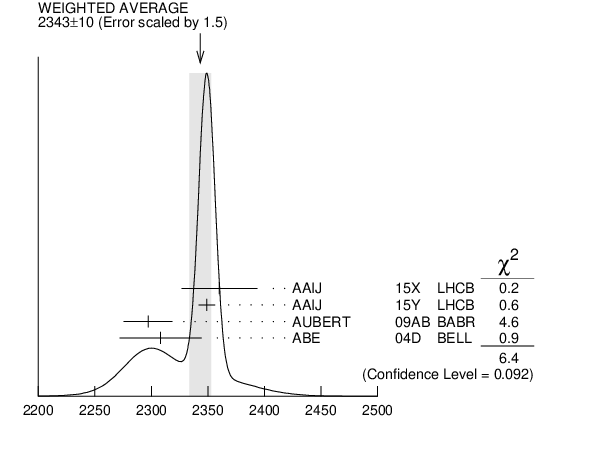${{\boldsymbol D}_{{0}}^{*}{(2300)}}$ MASS

VALUE (MeV) EVTS DOCUMENT ID TECN CHG  COMMENT
 $\bf{ 2343 \pm10}$ OUR AVERAGE  Error includes scale factor of 1.5. See the ideogram below.
$2360$ $\pm15$ $\pm30$ 1
 2015 X
LHCB + ${{\mathit B}^{0}}$ $\rightarrow$ ${{\overline{\mathit D}}^{0}}{{\mathit K}^{+}}{{\mathit \pi}^{-}}$
$2349$ $\pm6$ $\pm4$ 2
 2015 Y
LHCB + ${{\mathit B}^{0}}$ $\rightarrow$ ${{\overline{\mathit D}}^{0}}{{\mathit \pi}^{+}}{{\mathit \pi}^{-}}$
$2297$ $\pm8$ $\pm20$ 3.4k
 2009 AB
BABR 0 ${{\mathit B}^{-}}$ $\rightarrow$ ${{\mathit D}^{+}}{{\mathit \pi}^{-}}{{\mathit \pi}^{-}}$
$2308$ $\pm17$ $\pm32$
 2004 D
BELL 0 ${{\mathit B}^{-}}$ $\rightarrow$ ${{\mathit D}^{+}}{{\mathit \pi}^{-}}{{\mathit \pi}^{-}}$
• • We do not use the following data for averages, fits, limits, etc. • •
$2354$ $\pm7$ $\pm11$ 3
 2015 Y
LHCB + ${{\mathit B}^{0}}$ $\rightarrow$ ${{\overline{\mathit D}}^{0}}{{\mathit \pi}^{+}}{{\mathit \pi}^{-}}$
$2403$ $\pm14$ $\pm35$ 18.8k 4
 2004 A
FOCS + ${{\mathit \gamma}}$ A
$2407$ $\pm21$ $\pm35$ 9.8k 4
 2004 A
FOCS 0 ${{\mathit \gamma}}$ A
 1 From the Dalitz plot analysis including various ${{\mathit K}^{*}}$ and ${{\mathit D}^{**}}$ mesons as well as broad structures in the ${{\mathit K}}{{\mathit \pi}}$ ${\mathit S}{\mathrm -wave}$ and the ${{\mathit D}}{{\mathit \pi}}$ $\mathit S$- and ${\mathit P}{\mathrm -wave}$s.
 2 Modeling the ${{\mathit \pi}^{+}}{{\mathit \pi}^{-}}$ ${\mathit S}{\mathrm -wave}$ with the Isobar formalism.
 3 Modeling the ${{\mathit \pi}^{+}}{{\mathit \pi}^{-}}$ ${\mathit S}{\mathrm -wave}$ with the K-matrix formalism.
 4 Possibly the feed-down from another state.${{\mathit D}_{{0}}^{*}{(2300)}}$ MASS (MeV)
References:
 AAIJ 2015Y
PR D92 032002 Dalitz Plot Analysis of ${{\mathit B}^{0}}$ $\rightarrow$ ${{\overline{\mathit D}}^{0}}{{\mathit \pi}^{+}}{{\mathit \pi}^{-}}$ Decays
 AAIJ 2015X
PR D92 012012 Amplitude Analysis of ${{\mathit B}^{0}}$ $\rightarrow$ ${{\overline{\mathit D}}^{0}}{{\mathit K}^{+}}{{\mathit \pi}^{-}}$ Decays
 AUBERT 2009AB
PR D79 112004 Dalitz Plot Analysis of
 ABE 2004D
PR D69 112002 Study of ${{\mathit B}^{-}}$ $\rightarrow$ ${{\mathit D}^{**0}}{{\mathit \pi}^{-}}$ ( ${{\mathit D}^{**0}}$ $\rightarrow$ ${{\mathit D}}{}^{(*)+}$ ${{\mathit \pi}^{-}}$ ) Decays
PL B586 11 Measurement of Masses and Widths of Excited Charm Mesons ${{\mathit D}_{{2}}^{*}}$ and Evidence for Broad States